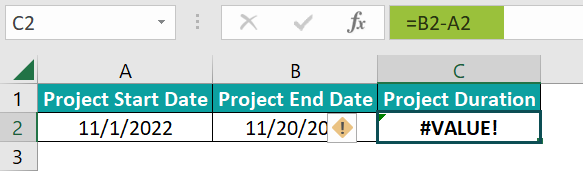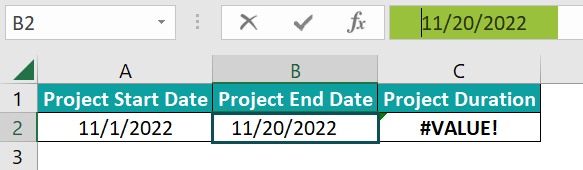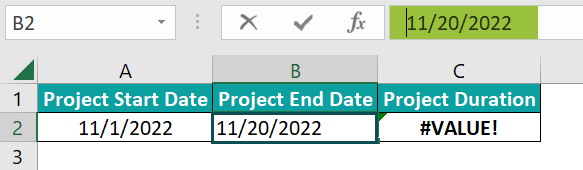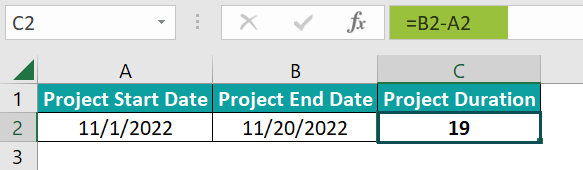# #VALUE! Error In ExcelArticle byExcelMojo Team## What Is #VALUE! Error In Excel?

The #VALUE! error in Excel is an error that occurs when the argument value supplied to a function is of the wrong data type or the function syntax is incorrect. Users can face the #VALUE! error when they supply texts as arguments to arithmetic formulas, refer to cells containing additional spaces, or enter a date as a text in a function.

For example, the following table contains sets of two values and descriptions stating the operations to perform using them.

The requirement is to apply the formulas in column D based on the given descriptions. And assume we have applied the required formulas in the target cells and obtained the below output.

The results show that the above scenario is a #VALUE error in Excel example.

As per rows 2 and 3 descriptions, the operations are arithmetic. But, as one of the input values in each row is a text, you get the VALUE error in Excel when dividing and subtracting the given numbers in the target cells D2 and D3, respectively.

In the case of row 4, the supplied date to EDATE Excel function does not have the correct date format, leading to the #VALUE! error as the output.

And in row 5, the ODD function syntax is incorrect, as the supplied argument value is a range instead of a number, thus leading to the #VALUE! error in Excel.

###### Key Takeaways
• The #VALUE! error in Excel occurs when you provide arguments of the wrong type to a function, or the function syntax is incorrect.
• Users will face the #VALUE! error in scenarios such as when they supply texts as arguments to arithmetic formulas or use references to cells containing additional spaces in functions.
• Suppose you require to remove the #VALUE! error, use the IF or IFERROR functions. These functions enable you to display a message or value in place of the #VALUE! error, according to your requirements.

### Scenarios Of Getting #VALUE! Error In Excel

In this section, we shall see the scenarios of getting the #VALUE! error.

We will better understand the #VALUE error in Excel meaning, which will help us fix it effectively.

#### VALUE Error Due To Text String In Formulas

Suppose a formula works only with numbers, but we supply non-number values to the formula as input. Then, executing it will result in the #VALUE! error in Excel.

For example, the below table contains a grocery list and each item’s price.

And the requirement is to find and display the total price of the items in the list in cell B7. Then, the steps are as follows:

• Step 1: Select the target cell B7, enter the below expression, and press Enter.

=B2+B3+B4+B5+B6

In the above example, the two items in rows 4 and 5 have their prices missing, with the displayed prices being text strings.

Thus, the above formula refers to cells containing numbers and text strings and adds the values to return the #VALUE! error. The error is because the expression performs an arithmetic operation that works only with numbers. But some input values, in this case, are text strings.

Solutions

Here are two ways of handling #VALUE error in Excel.

• Delete the strings from the source data

Suppose we delete the texts from cell range B4:B5 and apply the above arithmetic formula in the target cell B7. Then, the output will be:

The formula counts the empty cells as 0 to return the sum of the prices, \$1.01, \$3.21, \$0, \$0, and \$11.58 as \$15.8.

• Use Excel inbuilt functions

Excel’s built-in functions help in handling #VALUE error in Excel effectively, as they ignore text strings, cells with space characters, and empty cells.

For example, we can apply the Excel SUM function in the target cell B7 to determine the total price of groceries without deleting the strings from the source table.

• Step 1: Select the target cell B7, enter the SUM(), and press Enter.

=SUM(B2:B6)

In the above #VALUE error in Excel example, the SUM() ignores the texts in the specified cell range B2:B6. And it only adds the numbers to return the total grocery price as \$15.8.

#### Value Error Due To Incorrect Argument Type In Formulas

We can get the #VALUE! error in Excel when we supply argument values, of incorrect data types, to the function we wish to execute.

For example, the below table contains the inputs required to apply the MID Excel function in the target cell D2 to display the text “#VALUE! error”.

Suppose we supply the cell references in Excel to the specified values in cell range A2:C2 as the MID() arguments in cell D2, as explained below.

• Step 1: Select the target cell D2, enter the MID(), and press Enter.

=MID(A2,B2,C2)

Then the outcome is the #VALUE! error. The reason is that as per the MID() syntax, the second argument, start_num, should be a number or a formula returning a number. But in this case, the supplied start_num argument is the ‘#’ symbol.

Solutions

• Double-click the cell containing the expression to confirm the supplied arguments have the correct data type.

This option might help when you know the argument types the Excel function accepts.

• Click the expression in the Formula Bar to verify the function syntax and confirm the argument data type we require to supply to the function.
• Use the Error Checking option in the Formulas tab.
• Step 1: Choose the cell containing the formula and click FormulasError Checking to open the Error Checking window.

The Error Checking window shows the reason for the error, thus guiding us in correcting it.

We can also click the second option to view the calculation steps in the Evaluate Formula window.

Here, we can check the argument values we supplied to the function. And we can click Evaluate to determine the calculation step that results in the error.

Thus, the above methods show the issue that caused the #VALUE! error to occur. So, once we correct the source data in cell B2 by replacing the ‘#‘ symbol with the position of the first character to extract, which is 27, we get the required result.

On the other hand, if the entered formula is complex, we can select a section of the expression in the Formula Bar and click F9. Doing so enables us to see the calculation in the selected part and correct the formula in sections.

#### Value Errors Due To Incorrect Date Format

We may use Excel Date functions or formulas that include Date functions as part of the required calculations.

In such scenarios, supplying date values in the incorrect format can lead to the formula or function returning the #VALUE! error in Excel.

For example, the following table contains a few date values.

Suppose the requirement is to determine the total number of working days between the given start and end dates, excluding weekends and the specified holiday. And the target cell is D2.

Then, we can apply the NETWORKDAYS excel function in the target cell to obtain the required data.

• Step 1: Select the target cell D2, enter the NETWORKDAYS(), and press Enter.

=NETWORKDAYS(A2,B2,C2)

But the function returns the #VALUE! error, as the specified holiday in cell C2, does not have the appropriate date format.

Solutions

• Ensure the dates supplied as the function arguments are of the correct date format.

While one way is to be careful while entering the dates in the source table, the other is to use the DATE() to enter the date in a cell or formula. But to do so, we must know the DATE() syntax well.

The function accepts three mandatory arguments, year, month, and day, as inputs, with the three input values being numbers.

So, applying the DATE() in the above table to enter the holiday date in cell C2 will ensure the NETWORKDAYS() returns the correct output.

• Step 1: Select the target cell C2, enter the DATE(), and press Enter.
• Add a number, say, one, to the entered date to check if it results in the next date. The date will return an error if it does not have the required format.

For example, when we add one to the three given dates in the source table, the results are as follows.

• Step 1: Select the target cell A5, enter the following formula, and press Enter.

=A2+1

• Step 2: Using the fill handle, implement the formula in cell range B5:C5.

Since cell range A2:B2 contain dates in the proper format, adding one to the results in date values. But the cell C2 date value does not have the required format. And adding one to it results in an error, thus indicating it needs correction.

### Fixing #VALUE! Error Using IF Or IFERROR Functions

The scenarios explained in the previous section would have helped us understand the #VALUE error in Excel meaning effectively.

We shall now see how to fix the #VALUE! error in Excel using the IF or IFERROR functions with an example.

The below table contains a list of items, their total prices, and unit details.

Suppose we must determine the price per unit of each item and display the data in column D. Then, the following steps will fetch us the required data.

• Step 1: Select the target cell D2, enter the below expression, and press Enter.

=B2/C2

• Step 2: Using the fill handle, update the formula in cell range D3:D6.

Let us see the cell D6 expression to understand how the formula works. The formula divides the total price by the total units to return the price per unit. However, the formula gives the VALUE error in Excel when dividing the cell range B6:C6 data. The reason is that the cell C6 value is a text, and the formula performs an arithmetic operation that works only for numbers.

Likewise, rows 3 and 5 also have a text string as one of the given values, leading to the respective target cells’ formulas returning the #VALUE! error.

Solution

Let us add two columns to the above table to show how to use the IFERROR() or IF() to remove the #VALUE! error.

• Step 1: Select the target cell E2, enter the IFERROR(), and press Enter.

=IFERROR(B2/C2,”Data Not Available”)

• Step 2: Select the target cell F2, enter the IF(), and press Enter.

=IF(ISERROR(B2/C2),”Data Not Available”,B2/C2)

• Step 3: Select cells E2:F2 and implement the formulas in the cell range E3:F6 using the fill handle.

The IFERROR() checks if dividing the two given numbers returns an error. And if the output is an error, the function returns the specified message to display in place of the error. Otherwise, the IFERROR() return value is the quotient resulting from dividing the two numbers as the required price per unit of the corresponding item.

In the case of the IF(), the IF condition is the ISERROR(). It checks if dividing the two numbers results in error. So, if the division results in error, the ISERROR excel function returns TRUE. Thus, the IF condition holds, and the IF() returns the specified message to display in place of the error.

But if the ISERROR() output is FALSE, the IF() returns the quotient from dividing the two given numbers.

### Find All Cells With The #VALUE! Error

You can find the #VALUE! error in Excel using the Find & Select feature in the Home tab.

For example, the below table shows the results of the specified arithmetic operations.

Suppose the applied formulas return the #VALUE! error, and the requirement is to find cells containing the #VALUE! error. Then, the steps are as follows:

• Step 1: Click HomeFind & Select Find in the current spreadsheet containing the above table, or press Ctrl + F to access the Find and Replace window.
• Step 2: Click Options to access additional options.
• Step 3: Update the Find what field with #VALUE!, set the below settings, and click Find All.

The above settings will find the #VALUE! error in the current worksheet. But if we wish to locate it in the workbook, set the Within field as Workbook using the drop-down.

And once we click Find All, Excel finds and lists the cells containing the #VALUE! error.

Finally, we can click a cell from the list highlighted in the above image to select the cell in the worksheet.

But for highlighting all cells in the sheet containing the #VALUE! error, click on a cell in the list highlighted in the above image and press Ctrl + A. It will highlight all cells in the sheet containing the error value.

### Important Things To Note

• Ensure to supply only numbers, in the appropriate data formats, to formulas performing mathematical calculations. Otherwise, you can get the #VALUE! error in Excel.
• Check each argument type when supplying it to the required Excel function and verify the function syntax to avoid the #VALUE! error.
• Verify the date format when supplying the date as an argument to an Excel function. Otherwise, the function might return the #VALUE! error.
• Suppose you require to find the cells containing the #VALUE! error in a worksheet or workbook, use the Find and Replace feature.

1. What are the reasons to get the #VALUE! error in Excel?

The reasons to get the #VALUE! error in Excel are:
You supply text values to arithmetic formulas.
The supplied argument to a function or formula is a reference to a cell containing hidden or additional space characters.
The supplied date value to a function or formula is in the Text format.

2. Does a formula referencing a cell containing additional spaces return the #VALUE! error?

A formula referencing a cell containing additional spaces returns the #VALUE! error.

For example, the below table contains a project’s start and end dates. And you subtract the smaller date from the bigger date to determine the project duration in cell C2.However, the formula in the target cell gives the #VALUE! error as the output, as one of the input values, cell B2, contains additional leading spaces before the date.And once we remove the additional space characters, you will get the required output.

• Step 1: Double-click cell B2 and delete the three additional spaces from the cell or the Formula Bar.• Step 2: Press Enter or click outside cell B2 to get the required project duration.3. What happens when you do not execute an array formula using Ctrl + Shift + Enter keys?

When you do not execute an array formula using Ctrl + Shift + Enter keys, the formula output is the #VALUE! error.